# An Integrated Approach for Modeling Three-Phase Micro Hydropower Plants

An Integrated Approach for Modeling Three-Phase Micro Hydropower Plants

Achour El Hamdaouy* Issam Salhi Said Doubabi Najib Essounbouli Mohammed Chennani

Superior Technology School, Ibn Tofail University, B.P. 242, Kenitra 14090, Morocco

Laboratory of Electric Systems and Telecommunications, Cadi Ayyad University, BP 549, Av Abdelkarim Elkhattabi, Gueliz, Marrakesh 40000, Morocco

CReSTIC, Reims University, 9, rue de Québec B.P. 396, F-10026, Toyes cedex 10026, France

Corresponding Author Email:
a.elhamdaouy@uit.ac.ma
Page:
479-487
|
DOI:
https://doi.org/10.18280/ejee.210601
5 May 2019
|
Accepted:
9 August 2019
|
Published:
21 December 2019
| Citation

OPEN ACCESS

Abstract:

Micro hydropower plants (MHPPs) are often adopted to provide electricity to remote areas. To ensure the generation performance and power quality, the operators must have enough knowledge about the MHPP, especially its physical model. This paper proposes an integrated approach for modelling three-phase MHPPs in off-grid sites. The differential equations about the electrical operations and mechanical behaviors of the generator were fully integrated into our approach. The physical model thus established considers the behavior of different components of the target MHPP, such as the turbine and the generators, and helps to obtain the time-variation of all physical parameters of the MHPP. The validity of the integrated approach was verified through a simulation under three scenarios. Our research makes it possible to develop a physical model suitable for single and/or three-phase loads. The model can be easily adapted to any real configuration of hydropower plant.

Keywords:

renewable energy, micro hydropower plant, modelling, Pelton turbine, synchronous generator

1. Introduction

Nowadays, electrical energy demand is growing continuously. This is due to an unavoidable need for this vital energy, its serious depletion, and aberrant usage. Consequently, obtaining other electrical energy sources has become an indisputable necessity [1-4]. For this reason, renewable energy attracted the attention of the world, particularly hydro energy that has become an outstanding source to generate electricity all over the world . As a matter of fact, hydropower plants help limit toxic gases emissions by providing 19% of the world’s electricity . In addition, the hydropower has many other benefits it does not encounter the population displacement and environmental problems. In remote areas, hydropower plants are considered more efficient than other sources of renewable energy like wind or solar . In fact, solar cells convert about 10% to 12% of light energy directly to electric energy whereas hydro plants have an efficiency between 60% to 90% range .

At the national level, Morocco has significant hydraulic potential mainly in the mountainous regions [8, 9]. A hydropower plant is considered as a micro hydropower plant (MHPP) when a nominal generated power less than 200 kW . The MHPP can produce electricity from run-of-river to satisfy the locals’ needs . Hence, the emergence of the idea of installing MHPP in different mountainous areas, especially in isolated sites where it is difficult to be connected to the national grid. This electricity facilitates the local community’s main requirements for agricultural land irrigation. Moreover, it will enhance the living standards by fostering the educational level, hospital services, communications, conservation of agricultural products (fruits and vegetables) and providing the heat during winter.

Since the development of MHPP prototypes is costly [12-21], it has become necessary to develop mathematical models and use computer simulations in order to study the MHPP operation. The rationale behind this is to develop control approaches ensuring the expected performances. So, obtaining a model reflecting the MHPP real dynamic in the transient state as well as the steady-state is a necessity.

In the literature, most of the proposed MHPP models are based on transfer functions which are validated for a specific operating point. In other words, the transfer function based models do not reflect the MHPP overall operation, especially its nonlinearities. For example, the MHPP model, proposed in the scientific article , gives only the frequency evolution parameter around a specific operating point and uses linear transfer functions. However, developing a control system for large operating points is not considered. Although, a nonlinear MHPP model around several operating points was proposed in the research article . It is not based on differential equations describing the MHPP behavior. This model requires identification tests wherever a different MHPP is involved. The frequency regulation was studied in the scientific article  without simulation and working directly on a prototype. The simulation might give more opportunities to design suitable robust controllers. An MHPP mathematical model for a single-phase MHPP was presented in the research article . In this work, the developed model gives only the frequency evolution and just takes into consideration resistive loads. A scheme of sliding mode control by model order reduction for load control problem of MHPP was discussed in the scientific article . This scheme was tested under isolated and connected grid modes. The proposed schemes are based on transfer functions and devote only the frequency evolution. In the research article , a multi-source power system approach, using teaching-learning optimization algorithm for automatic generation controller of the power system, was suggested. They use 2-degree freedom of proportional-integral-derivative controller. In addition, the multi-source model combines several source models such as thermal, hydro and gas powers which are modeled by transfer functions. The approach was compared with some published approaches. The software application platform, developed in the research article , is contained a platform component library that is based on linear equations. These equations cannot correctly simulate the behavior under different conditions of MHPP and so, it has limitations. Considering the previous works, any realistic models for three-phase MHPP, which reflect all physical phenomena such as reactive power and converters impact, are not suggested.

The MHPP models conception must consider all kind of loads. In most sites where MHPPs are likely to be installed, locals use generally single-phase loads, inductive and resistive type such as lamps, televisions and small engines except for those who use three-phase pumps for irrigation or different equipments for commercial activities. In the literature, the models consider resistive loads which are generally characterized by their nominal power. These models do not have the flexibility to study the connected loads random effect and all kind of loads.

This paper presents a modeling approach to describe the three-phase MHPP overall operation for all operating points range. In fact, it is based on the electrical and the mechanical differential equations expressing the behavior of the constituting MHPP components. These equations illustrate the MHPP nonlinear behavior. The developed model could be used by researchers interested in the MHPP control, to develop new advanced controllers. The model gives also the opportunity to improve the power quality in remote sites, especially when the proposed three-phase MHPP model is used in closed-loop. Besides, it might be useful for academic and educational purposes, so that students can easily understand the MHPP real operation. Among the strengths of our model is that it provides the temporal evolution of all MHPP physical parameters (different currents, voltages, frequency, powers, torques, etc.). In addition, using the developed model, different kind of loads can be connected and tested (three-phase, single-phase resistive and/or inductive loads, etc.). Therefore, it should be integrated in Matlab/Simulink.

There are no specific international standards about isolated electrical systems; however, the power should be similar to that of the international standards , because the consumers’ equipment of both isolated and interconnected grids requires the same power quality to operate. In remote sites, loads are generally resistive with an inductive effect such as three-phase pumps and small engines, etc. These loads require a reactive power for their operation. The need to improve and manage this reactive power in these sites, using an efficient way (reactive power compensation) is suitable. In this context, the developed MHPP model could be used by researchers in the closed-loop to develop advanced controllers for enhancing the power factor. In addition, the developed model gives more chances to adapt and to propose frequency controllers for all MHPP control techniques such as speed-flow, load-frequency, and mixed control. Moreover, the proposed approach devotes the feasibility and the simplicity to coordinate the hydropower plant with other sources, like wind and solar, etc. After coordination, the researchers have the possibility to regulate different parameters, since the integrated approach permits getting all physical parameters evolution versus time. Considering all these beneficial points, the developed MHPP model is considered as an added value for renewable energy.

The most suitable turbine for such configuration is the Pelton turbine which is generally used for high waterfalls and can operate over a wide range of water flow level . The flow rate is adjustable by means of a movable needle which moves manually or by an electric servo-motor . On the other hand, the three-phase synchronous generator is more appropriate, for several advantages; it presents a very satisfactory efficiency (close to 99%) and its size is relatively small compared to the generated power [5, 29].

2. Model of the Hydraulic Turbine

The MHPP operation principle consists of transforming the hydraulic energy into electrical energy. Through a water tunnel, the water reaches a surge tank that supplies a penstock characterized by its height. At the output of this latter, the water rotates a hydraulic turbine which drives a synchronous generator. The generator produces electricity that feeds loads which can be composed from three or/and single-phase loads. Figure 1 shows the various components of the constituting hydropower plants.

## 1.jpg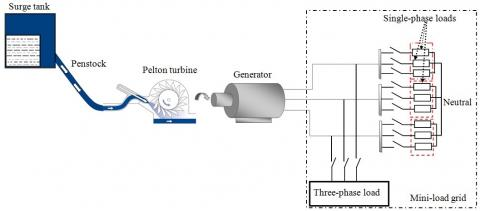Figure 1. Hydropower plant components

The Pelton turbine consists of buckets set on a circular disk periphery and one or many nozzles which strike the buckets by water jets. The water flow can be adjusted by a mobile needle inside the nozzle that is usually driven by an electric servo-motor which must be relatively slow to minimize water hammers effect .

The hydraulic power is given by the expression (1) :

$P_{t}=\rho g Q_{t} H_{t}$ (1)

with: $\rho$ is the water’s density (kg m-3), Qt  is the water flow (m3 s-1), Ht is the effective waterfall height (m) and g is the gravity acceleration (m s-2).

In the Pelton turbine case, the hydraulic power expression becomes :

$P_{t}=\rho Q_{t} U\left(V_{1}-U\right)(1+m \cos \beta)$ (2)

with: U is the turbine’s drive speed, V1 is the water’s speed in the contact of the jet with the buckets, m is the ratio of V1 and V2 and $\beta$  is the angle between $\overrightarrow{V_{1}}$ and $\overrightarrow{V_{2}}$.

To make the equations easy to interpret, reduced quantities "per unit system" (pu) have been adopted for the model, and the international system (IS) units have been adopted for the physical quantities.

Based on Eq. (2), the Pelton turbine torque is given by the expression (3):

$C_{t}=\rho Q_{t} \frac{U}{\Omega_{t}}\left(V_{1}-\frac{D_{t}}{2}\right)(1+m \cos \beta)$ (3)

with: $\Omega_{t}$  is the angular velocity of the generator (rad s-1) and Dt is the diameter of the turbine (m).

The jet velocity at the penstock exit expression is given by the Eq. (4):

$\mathrm{V}_{1}=\sqrt{2 \mathrm{gH}_{\mathrm{t}}}$ (4)

The turbine speed expression in (pu) becomes:

$\gamma_{\mathrm{t}}=\sqrt{\mathrm{h}_{\mathrm{t}}}$ (5)

with: h is the waterfall height (pu).

The turbine torque expression using the reduced quantities becomes:

$c_{\mathrm{t}}=\frac{q_{\mathrm{t}}\left(\sqrt{\mathrm{h}_{\mathrm{t}}}-\mathrm{k}_{\mathrm{t}} \mathrm{n}_{\mathrm{t}}\right)}{\left(1-\mathrm{k}_{\mathrm{t}}\right)}$ (6)

where,

$\mathrm{k}_{\mathrm{t}}=\frac{\mathrm{D}_{\mathrm{t}} \Omega_{\mathrm{tn}}}{2 \mathrm{V}_{\mathrm{1n}}}$ (7)

with: $q_{t}$ is the water flow $(p u), n_{t}$ is the turbine speed $(p u), c_{t}$ is the turbine torque (pu), $V_{1 n}$ is the nominal speed of the jet

$\left.\left(\mathrm{m} \mathrm{s}^{-1}\right) \text { and } \Omega_{t n} \text { is the nominal speed of the turbine (rad } \mathrm{s}^{-1}\right) .$

3. Model Representing the MHPP

Using a single-phase generator is more expensive in kilowatt (kW) compared to a three-phase generator . For this reason, it is the most recommended generators to be used in MHPP for isolated sites. The three-phase synchronous generator can be modeled by different methods such as the linear state representation and the equivalent electrical circuit in the reference frame d-q. The linear state representation presents a disadvantage, bearing in mind, the machine speed involved in the state matrix [31, 32]. This assumes that the speed remains steady along with the simulation, whereas in the MHPP case, this speed depends on the operating conditions. The equivalent electrical circuit helps to reflect the MHPP overall behavior. Therefore, this paper uses the differential equations which describe the generator electrical operation as well as those describing the mechanical behavior.

In the literature, some research works use the synchronous generators blocks are provided by the toolbox «Sim Power System» of Matlab/Simulink . However, these models are not suitable for the following tests: overload/load discharge, short-circuit, etc.

3.1 The generator’s electrical equations

The synchronous generator is a rotating electric machine directly coupled with the hydraulic turbine. It consists of a rotating part named the rotor and a fixed part named the stator. The rotor has a field winding (f) (inductor) and absorbs the hydraulic power. The stator has three armatures windings (a, b and c) and is connected in parallel with the mini-load grid presented in Figure 1. Figure 2 shows the synchronous generator view without dampers. A "receiver" convention is adopted for the rotor and a "generator" convention is adopted for the stator. The generator voltages can be described in three-phase reference by the Eqns. (8) :

\begin{aligned} \mathrm{v}_{\mathrm{a}} &=-\mathrm{r}_{\mathrm{s}} \mathrm{i}_{\mathrm{a}}+\frac{\mathrm{d} \varphi_{\mathrm{a}}}{\mathrm{dt}} \\ \mathrm{v}_{\mathrm{b}} &=-\mathrm{r}_{\mathrm{s}} \mathrm{i}_{\mathrm{b}}+\frac{\mathrm{d} \varphi_{\mathrm{b}}}{\mathrm{d} \mathrm{t}} \\ \mathrm{v}_{\mathrm{c}} &=-\mathrm{r}_{\mathrm{s}} \mathrm{i}_{\mathrm{c}}+\frac{\mathrm{d} \varphi_{\mathrm{c}}}{\mathrm{d} \mathrm{t}} \\ \mathrm{v}_{\mathrm{f}} &=\mathrm{r}_{\mathrm{f}} \mathrm{i}_{\mathrm{f}}+\frac{\mathrm{d} \varphi_{\mathrm{f}}}{\mathrm{dt}} \end{aligned} (8)

where, ia,b,c  are the armature currents (A), $\varphi_{a, b, c}$ are the total armature flux (wb), $\varphi_{\mathrm{f}}$ is the total main field flux (A), rf is the main field resistance (Ω), rs is the stator resistance (Ω) and vf, if are the main field excitation voltage (V) and the main field current (A), respectively.

## 2.jpgFigure 2. Synchronous generator view without dampers

Stator and rotor flux terms are functionally related to the rotor angle ($\theta$) which varies with time at the rotor rotation speed rate. These elements have computational problems to solve direct phase quantities. To achieve, the use of the Park transformation to shift from a fixed reference point linked to the stator towards a rotating reference frame linked to the rotor is recommended. The result of this transformation, called reference frame d-q, is a mathematical tool for describing a generator behavior using equations with fixed coefficients. Hence, the generator analytical study becomes relatively simple in the reference frame d-q . The Eq. (9) shows the used Park transformation matrix ( Park ).

$\left[P_{a r k}\right]=\sqrt{\frac{2}{3}}\left[\begin{array}{ccc}{\cos \theta} & {\cos \left(\theta-\frac{2 \pi}{3}\right)} & {\cos \left(\theta+\frac{2 \pi}{3}\right)} \\ {-\sin \theta} & {-\sin \left(\theta-\frac{2 \pi}{3}\right)} & {-\sin \left(\theta+\frac{2 \pi}{3}\right)} \\ {\frac{1}{\sqrt{2}}} & {\frac{1}{\sqrt{2}}} & {\frac{1}{\sqrt{2}}}\end{array}\right]$ (9)

The stator transformed voltages, currents and flux are given by the Eqns (10):

\begin{aligned}\left[\mathrm{V}_{\mathrm{qd} 0}\right] &=\left[\mathrm{P}_{\mathrm{ark}}\right]\left[\mathrm{V}_{\mathrm{abc}}\right] \\\left[\mathrm{i}_{\mathrm{qd} 0}\right] &=\left[\mathrm{P}_{\mathrm{ark}}\right]\left[\mathrm{i}_{\mathrm{abc}}\right] \\\left[\varphi_{\mathrm{qd} 0}\right] &=\left[\mathrm{P}_{\mathrm{ark}}\right]\left[\varphi_{\mathrm{abc}}\right] \end{aligned} (10)

where, Vd, Vq are the direct and the transverse voltages (V) respectively; id , iq are the direct and the transverse currents (A) respectively and $\varphi_{\mathrm{d}}$, $\varphi_{q}$ are the direct and the transverse total flux (wb) respectively.

The synchronous generator model is defined in the reference frame d-q. The main assumptions taken into consideration for modeling are:

• The magnetic circuit saturation, hysteresis and eddy currents are assumed negligible. The capacitive coupling between windings are assumed negligible.
• The stator and the main field resistances of generator are invariant with respect to changes in temperature.
• The magnetomotive forces of armature windings are sinusoidal distribution.
• The leakage inductances and the leakage flux are assumed negligible.

Finally, the stator voltages and flux of three-phase synchronous generator in the reference frame d-q are written by the Eqns. (11) and (12), respectively.

\begin{aligned} \mathrm{V}_{\mathrm{d}}=&-\mathrm{r}_{\mathrm{s}} \mathrm{i}_{\mathrm{d}}+\frac{\mathrm{d} \varphi_{\mathrm{d}}}{\mathrm{dt}}-\mathrm{w}_{\mathrm{e}} \varphi_{\mathrm{q}} \\ \mathrm{V}_{\mathrm{q}}=&-\mathrm{r}_{\mathrm{s}} \mathrm{i}_{\mathrm{q}}+\frac{\mathrm{d} \varphi_{\mathrm{q}}}{\mathrm{dt}}+\mathrm{w}_{\mathrm{e}} \varphi_{\mathrm{d}} \\ & \mathrm{V}_{\mathrm{f}}=\mathrm{r}_{\mathrm{f}} \mathrm{i}_{\mathrm{f}}+\frac{\mathrm{d} \varphi_{\mathrm{f}}}{\mathrm{dt}} \end{aligned} (11)

\begin{aligned} \varphi_{\mathrm{d}}=&-\mathrm{L}_{\mathrm{d}} \mathrm{i}_{\mathrm{d}}+\mathrm{m}_{\mathrm{sf}} \mathrm{i}_{\mathrm{f}} \\ & \varphi_{\mathrm{q}}=-\mathrm{L}_{\mathrm{q}} \mathrm{i}_{\mathrm{q}} \\ \varphi_{\mathrm{f}}=& \mathrm{L}_{\mathrm{f}} \mathrm{i}_{\mathrm{f}}-\mathrm{m}_{\mathrm{sf}} \mathrm{i}_{\mathrm{d}} \end{aligned} (12)

with: we is the electrical speed (rad.s-1), msf is the mutual inductance between the field winding and d-axis stator winding (wb), Ld, Lq are the inductances of the d-axis stator winding and q-axis stator winding (H) respectively and Lf is the inductance of the main field winding (H).

Using the stator voltages and flux equations, the generator equivalent electrical circuit can be drawn, as it’s shown in Figure 3. This circuit can be used to simulate the electrical behavior of the generator.

## 3.jpgFigure 3. Electrical behavior of the generator using the equivalent electrical circuit in the reference frame d-q

3.2 Mechanical behavior

The synchronous generator modeling will not be completed without the electromagnetic torque expression . The electromagnetic torque is given by the expression (13):

$T_{e l e}=P\left[\varphi_{d} i_{q}-\varphi_{q} i_{d}\right]$ (13)

with: P is the machine’s poles number.

To illustrate the rotation speed, the synchronous generator model must incorporate the fundamental principle of dynamics, linking different torques to the mechanical rotor speed which is written as the Eq. (14):

$J_{\Delta} \frac{d}{d t}\left(w_{m}\right)+f_{v} w_{m}=T_{m e c h}-T_{s}-T_{e l e}$ (14)

with: $J_{\Delta}$ combines inertia moment of both the generator and the hydraulic turbine (kg m2), Wm is the mechanical rotor speed (rad s-1), Ts  is the sec friction torque (N m), fv is the viscous friction coefficient (N m/rad s-1) and Tmech  is the mechanical torque (N m).

3.3 Development of the generator model

The proposed model generates the three-phase voltage and creates the three terminals va , vb, and vc on which three/single-phase loads of mini-load grid can be connected.

## 4.jpg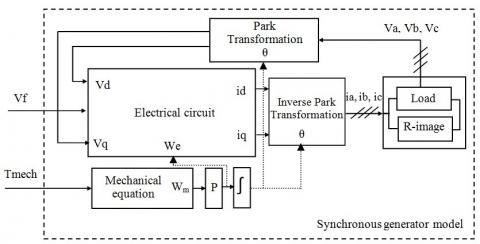Figure 4. Schematic diagram of the three-phase generator

The generator model, proposed in Figure 4, is characterized by two inputs: the mechanical torque ( Tmech) and excitation voltage ( vf ). The mechanical torque is the mechanical equation input for generating the electrical speed and the mechanical speed which will be useful for direct Park transformation blocks, inverse Park transformation and equivalent electrical circuit. The excitation voltage goal is generating the excitation current ( if ). Thus, creating static electromotive forces is due to flux variations and electrodynamics forces based on the pulses obtained by the mechanical equation. Afterwards, the equivalent electrical circuit provides the currents id  and iq characterizing the inputs of the inverse Park transformation block to generate the three-phase currents ( ia, ib , and ic ). The use of three resistances "R-image" which are equal to 106Ω provides the three-phase voltages ( va , vb, and vc ). Finally, the equivalent electrical circuit contains voltages ( vd  and vq ) which are the generator output voltages, but they are used as feedback to perform the correct creation of the currents id  and iq .

According to the Figure 4, the proposed generator model is characterized by two inputs (mechanical torque and excitation voltage) and three outputs (three voltage terminals ( va, vb , and vc )) in order to ensure the conversion of the mechanical energy to electricity. The model is suitable for all kind of loads single or/and three-phase which can be connected with the proposed model via the terminals.

3.4 Development of the MHPP model

To develop a global MHPP model shown in Figure 5, the previously developed models should be incorporated together. Interactions between different model blocks give the MHPP overall behavior and allow extracting the maximum information related to the MHPP operation (under all working conditions).

## 5.jpg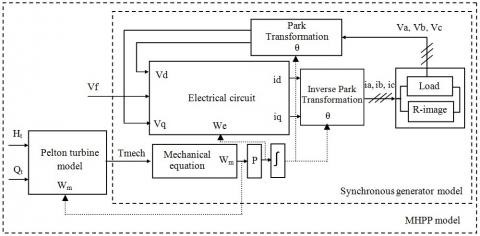Figure 5. Schematic diagram of the proposed MHPP model

4. Implementation and Simulation Results

Figure 6 shows the obtained global diagram Simulink model of the three-phase MHPP. The model was implemented using Matlab/Simulink 2010a, with Ode1 solver and running with a sample time equal to 10 . The electrical and mechanical parameters values of the used synchronous generator are shown in Table 1.

## 6.jpgFigure 6. Proposed Simulink model of a three-phase MHPP

Table 1. Three-phase generator parameters values

 Electrical parameters values: rs (Ω) rf (Ω) Ld (H) Lq (H) Lf (H) msf (H) 34.8618 6.635 0.4159 0.4159 0.0785 0.3841 Mechanical parameters values: Cs (N m) fv (N m/rad.s-1) $\mathrm{J}_{\Delta}$ (kg m2) P 0.6852 0.0025 0.005 2 Nominal values of the three-phase generator: Power Pn 270 W Frequency fn 50 Hz Voltage Un 400V Speed Nn 1500tr min-1 Current In 0.4A Power factor cos (φ) 0.7

4.1 Scenario 1: Load variations effects on the MHPP electrical quantities

The load variations effect on the frequency is a test among classic validation tests generally used in the literature. It’s based on connecting or disconnecting a load when the MHPP reaches its steady-state. The first scenario test is to run the hydropower plant with a nominal balanced three-phase load (270W) on the three phases and to connect, at the instant t=3s, three-phase resistive load constituting 25% of the rated load. After 10s, the same load is disconnected. Figure 7 presents the three-phase voltage line to line waveforms ( $U_{a b}, U_{b c},$ and $U_{c a}$ ) in the load terminals. Figure 8 shows the three-phase current waveforms absorbed by the load. Figures 9 and 10 show respectively the evolution of the frequency and the excitation current.

From figures 7 and 8, it can be seen when the MHPP is overloaded, the consumed current increases and the output voltage drops down. And when the same load is discharged, the absorbed current decreases and the output voltage return to their nominal values. According to the obtained curve of the Figure 9, as one can clearly see that the frequency evolution drops down (45.9Hz) when applying an overload of 25% and return to the nominal value (50 Hz) when the same connected load is disconnected. The results, illustrated in Figure 10, show a constant excitation current that equal its nominal value. These obtained results show a similar behavior compared to a real MHPP [16, 23].

## 7.pngFigure 7. Simulation waveforms of the three-phase voltage line to line versus time. (a) Before and after applying a load. (b) Zoom on the rated steady-state. (c) Zoom on the three-phase voltage line to line after applying an overload

## 8.pngFigure 8. Simulation waveforms of the three-phase current absorbed by the load versus time. (a) Before and after applying a load. (b) Zoom on the rated steady-state. (c) Zoom on the three-phase current absorbed by loads after applying an overload

## 99.png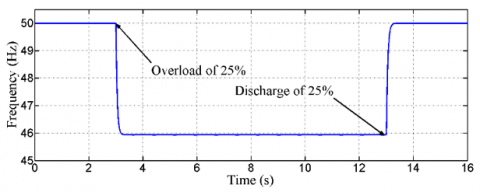Figure 9. Simulation frequency variation versus time when applying an overload followed by a load discharge

## 1010.pngFigure 10. Simulation of a load variation effect on the excitation current

Figures 7(b) and 8(b) respectively show a zoom on the three-phase voltage line to line waveforms and the three-phase current waveforms in the nominal steady-state. From these curves, as one can clearly see that their shapes are sinusoidal with a period equal to 0.02 s, have a phase shift of $\frac{2 \pi}{3}$  between two successive phases and root-mean-square (RMS) values equal to the nominal values.

It should be noted that all these simulation tests were performed in open-loop (without using any controller).

4.2 Scenario 2: Highlighting the nonlinear behavior of the MHPP

At the beginning of this scenario, the MHPP is driven at its nominal speed and nominal excitation, where the turbine flow rate and the effective waterfall height are constant. Afterwards, different load variations were made (overloads and load discharges of different values). The result corresponding to 4 different overload variation values is illustrated in Figure 11. Loads connections were applied at the instant t=1s. Similarly, Figure 12 illustrates the result for different load discharge values at the instant t=1s. According to figures 11 and 12 and reasoning on a one load variation (45%) as an example, it is clear that the hydropower plant is a nonlinear system. Indeed, for the same load variation (∆P), the frequency variation (∆f) is different between the load discharge and the overload: ∆f=10.5FigureHz for load discharge and ∆f=6.73Hz for overload. Table 2 provides a qualitative assessment of the resulting frequency variations for the applied load variations.

The non-linearity is also demonstrated by analyzing the frequency variations from different ∆P in the load discharge case. For example, ∆P=5% gives a ∆f=0.91Hz while ∆P=45%=5%*9 gives ∆f=10.59Hz instead of ∆f=8.19Hz=0.91Hz*9. This behavior is completely normal by comparing this result with recent research publications [16, 17, 23, 35]. The MHPP modeling with experimental validation, developed by the published article , has a strong non-linearity system and the frequency varies significantly with load variations. This recently published result was validated using an MHPP laboratory prototype which consists of a Pelton hydraulic turbine and a single-phase synchronous generator. According to the research article  and the present developed approach, the frequency evolution versus time behaviors, when applying different overloads and load discharges, is similar. In addition, the suggested MHPP model is a powerful tool, as the user could get all physical parameters evolution versus time, while the major existing models in the literature give only single parameter evolution: frequency around a specific operating point. It is concluded that the developed model is faithful to the MHPP real behavior.

## 1111.png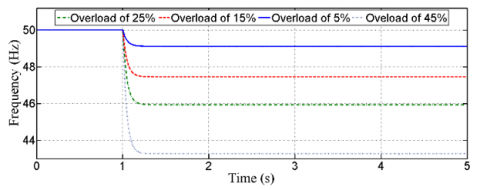Figure 11. Simulation frequency evolution versus time when applying different overloads

## 1212.png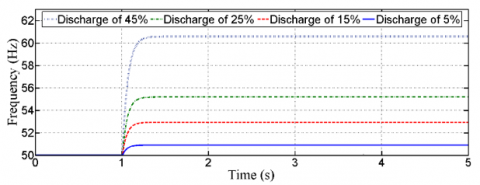Figure 12. Simulation frequency evolution versus time when applying different load discharges

Table 2. Qualitative assessment of simulation frequency variations resulting from load discharge/overload according to different values of ∆P

 ∆P 5% 15% 25% 45% |∆f| after load discharge 0.91 2.93 5.19 10.59 |∆f| after overload 0.88 2.55 4.06 6.73

4.3 Scenario 3: Test of unbalance load between the generator’s phases

In remote sites, the loads are generally single-phase. Thus, the third scenario consists of operating the MHPP, during a steady-state, with a nominal resistive three-phase load (270W). After that, at the instant t=1s, a single-phase load of 50W is connected to phase A only. This allows creating an unbalanced load into the three-phase generator terminals. The waveforms, illustrated in Figure 13, show the generator three-phase current waveforms before and after the unbalanced load. Figure 14(a) shows the resulting frequency evolution. The obtained result shows the appearance of an unbalanced current between phases. This unbalance generates torque pulsation in the generator that leads to mechanical vibration and acoustic noise . Figure 14(b) illustrates a zoom on the rated steady-state and justified the torque pulsation presence. Thus, the proposed model makes it possible to simulate the MHPP operation even in case of an unbalanced load.

## 1313.png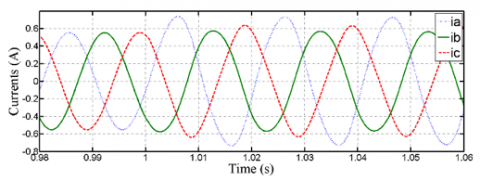Figure 13. Simulation waveforms of the three-phase current versus time before and after the use of unbalanced load

## 1414.png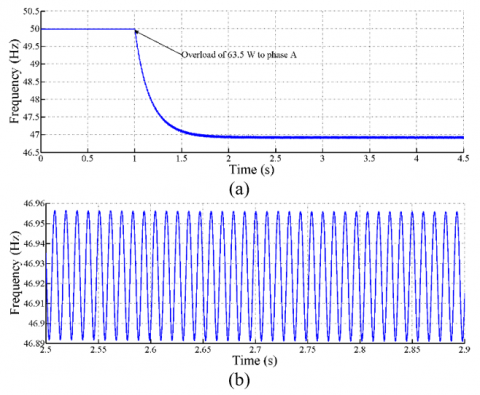Figure 14. Simulation frequency evolution versus time. (a) Before and after applying an overload to phase A. (b) Zoom on the rated steady-state

5. Conclusions

In this study, modeling of three-phase MHPP was presented. The model includes two sub-models, the Pelton turbine model and the three-phase synchronous generator model. And three scenarios tests were performed using the MHPP model. These scenarios show the performances and the flexibility of the proposed model to provide the temporal evolution of several MHPP parameters under all kind of MHPP operation conditions. The first scenario illustrates the load variations effect on the MHPP electrical quantities. The second scenario highlights the non-linear character of MHPP using load discharge and overload tests. The last scenario demonstrates the developed model flexibility to connect different kind of loads. This test demonstrates the feasibility to simulate the MHPP even in the case of an unbalance. Besides, simple identification methods can be used to adapt this model to any real hydropower plant configuration. The authors have as prospects to improve efficiency-cost for MHPP exploitation and to ensure the electricity quality continuously as the produced voltages characteristics remain constant (frequency and RMS), and to propose a control system to maintain these characteristics regardless of the random load variations.

References

 Berrada, A., Bouhssine, Z., Arechkik, A. (2019). Optimisation and economic modeling of micro hydropower plant integrated in water distribution system. Journal of Cleaner Production, 232(2): 877-887. https://doi.org/10.1016/j.jclepro.2019.06.036

 Sahoo, S.K. (2016). Renewable and sustainable energy reviews solar photovoltaic energy progress in India: A review. Renewable and Sustainable Energy Reviews, 59: 927-939. https://doi.org/10.1016/j.rser.2016.01.049

 Canales, F.A., Beluco, A., Mendes, C.A.B. (2015). A comparative study of a wind hydro hybrid system with water storage capacity: conventional reservoir or pumped storage plant. Journal of Energy Storage, 4: 96-105. https://doi.org/10.1016/j.est.2015.09.007

 Salimi, A.A., Karimi, A., Noorizadeh, Y. (2019). Simultaneous operation of wind and pumped storage hydropower plants in a linearized security-constrained unit commitment model for high wind energy penetration. Journal of Energy Storage, 22: 318-330. https://doi.org/10.1016/j.est.2019.02.026

 Dursun, B., Gokcol, C. (2011). The role of hydroelectric power and contribution of small hydropower plants for sustainable development in Turkey. Renewable Energy, 36(4): 1227-1235. https://doi.org/10.1016/j.renene.2010.10.001

 Laghali, J.A., Mokhlis, H., Bakar, A.H.A., Hasmaini, M. (2013). A comprehensive overview of new designs in the hydraulic, electrical equipments and controllers of mini hydro power plants making it cost effective technology. Renewable Energy, 20: 279-293. https://doi.org/10.1016/j.rser.2012.12.002

 Khan, A.A., Khan, A.A., Zahid, M., Rizwan, R. (2013). Flow acceleration by converging nozzles for power generation in existing canal system. Renewable Energy, 60: 548-552. https://doi.org/10.1016/j.renene.2013.06.005

 Salhi, I., Chennani, M., Doubabi, S., Ezziani, N. (2008). Modeling and regulation of a micro hydroelectric power plant. IEEE International Symposium on Industrial Electronics, Cambridge, UK, pp. 1639-44. https://doi.org/10.1109/ISIE.2008.4677235

 Alhamwi, A., Kleinhans, D., Weitemeyer, S., Vogt, T. (2015). Moroccan National Energy Strategy reviewed from a meteorological perspective. Energy Strategy Reviews, 6: 39-47. https://doi.org/10.1016/j.esr.2015.02.002

 Ghadimi, A.A., Razavi, F., Mohammadian, B. (2011). Determining optimum location and capacity for micro hydropower plants in Lorestan province in Iran. Renewable and Sustainable Energy Reviews, 15(8): 4125-4131. https://doi.org/10.1016/j.rser.2011.07.003

 Ranjitkar, G., Huang, J., Tung, T. (2006). Application of micro-hydropower technology for remote regions. IEEE EIC climate change technology, Ottawa, Canada. https://doi.org/10.1109/EICCCC.2006.277207

 Márquez, J.L., Molina, M.G., Pacas, J.M. (2010). Dynamic modeling, simulation and control design of an advanced micro hydro power plant for distributed generation applications. International Journal of Hydrogen Energy, 35(11): 5772-5777. https://doi.org/10.1016/j.ijhydene.2010.02.100

 Guo, W., Yang, J., Chen, J., Yang, W., Teng, Y., Zeng, W. (2015). Time response of the frequency of hydroelectric generator unit with surge tank under isolated operation based on turbine regulating modes. Electric Power Components and Systems, 43(20): 2341-2355. https://doi.org/10.1080/15325008.2015.1082681

 Vallet, M., Munteanu, I., Bratcu, A.I., Bacha, S., Roye, D. (2012). Synchronized control of cross-flow-water-turbine-based twin towers. Renewable Energy, 48: 382-391. https://doi.org/10.1016/j.renene.2012.05.013

 Guo, W., Yang, J., Yang, W., Chen, J., Teng, Y. (2015). Regulation quality for frequency response of turbine regulating system of isolated hydroelectric power plant with surge tank. Electrical Power and Energy Systems, 73: 528-538. https://doi.org/10.1016/j.ijepes.2015.05.043

 Salhi, I., Doubabi, S., Essounbouli, N., Hamzaoui, A. (2014). Frequency regulation for large load variations on micro-hydro power plants with real-time implementation. Electrical Power and Energy Systems, 60: 6-13. https://doi.org/10.1016/j.ijepes.2014.02.029

 Raja Singh, R., Anil Kumar, B., Shruthi, D., Panda, R., Thanga Raj, C. (2018). Review and experimental illustrations of electronic load controller used in standalone Micro-Hydro generating plants. Engineering Science and Technology, and International Journal, 21(5): 886-900. https://doi.org/10.1016/j.jestch.2018.07.006

 Khan, M.R.B., Pasupuleti, J., Jidin, R. (2018). Load frequency control for mini-hydropower system: A new approach based on self-tuning fuzzy proportional-derivative scheme. Sustainable Energy Technologies and Assessment, 30: 253-262. https://doi.org/10.1016/j.seta.2018.10.013

 Saxena, S. (2019). Load frequency control strategy via fractional-order controller and reduced-order modeling. Electrical Power and Energy Systems, 104: 603-614. https://doi.org/10.1016/j.ijepes.2018.07.005

 Xu, Y.P. (2017). A study of hydropower generation process control based on fuzzy control theory. European Journal of Electrical Engineering, 19(3-4): 167-179. https://doi.org/10.3166/EJEE.19.167-179

 Guo, B.Q., Xu B.B., Chen D.Y., Ye W., Guo P.C., Luo X.Q. (2018). Dynamic modeling and energy distribution analysis in a hydroelectric generating system considering the stochastic turbine flow. Electrical Power and Energy Systems, 103: 611-621. https://doi.org/10.1016/j.ijepes.2018.06.032

 Hanmandlu, M., Goyal, H. (2008). Proposing a new advanced control technique for micro hydro power plants. Electrical Power and Energy Systems, 30(4): 272-282. https://doi.org/10.1016/j.ijepes.2007.07.010

 Salhi, I., Doubabi, S., Essounbouli, N., Hamzaoui, A. (2010). Application of multi-model control with fuzzy switching to a micro hydro-electrical power plant. Renewable Energy, 35(9): 2071-2079. https://doi.org/10.1016/j.renene.2010.02.008

 Şerban, I., Marinescu, C. (2011). Aggregate load-frequency control of a wind-hydro autonomous microgrid. Renewable Energy, 36(12): 3345-3354. https://doi.org/10.1016/j.renene.2011.05.012

 Qian, D.W., Tong, S.W., Liu, X.J. (2015). Load frequency control for micro hydro power plants by sliding mode and model order reduction. Automatica, 56(3): 318-330. https://doi.org/10.7305/automatika.2015.12.816

 Sahu, R.K., Panda, S., Rout, U.K., Sahoo, D.K. (2016). Teaching learning based optimization algorithm for automatic generation control of power system using 2-DOF PID controller. Electrical Power and Energy Systems,  77: 287-301. https://doi.org/10.1016/j.ijepes.2015.11.082

 Garrido, J., Zafra, A., Vázquez, F. (2009). Object oriented modelling and simulation of hydropower plants with run-of-river scheme: A new simulation tool. Simulation Modelling Pratice and Theory, 17: 1748-1767. https://doi.org/10.1016/j.simpat.2009.08.007

 Cobb, B.R., Sharp, K.V. (2013). Impulse (Turgo and pelton) turbine performance characteristics and their impact on pico-hydro installations. Renewable Energy, 50: 959-64. https://doi.org/10.1016/j.renene.2012.08.010

 Haidar, A.M.A., Senan, M.F.M., Noman, A., Radman T. (2012). Utilization of pico hydro generation in domestic and commercial loads. Renew Sustain Energy Rev, 16(1): 518-524. https://doi.org/10.1016/j.rser.2011.08.017

 Derakhshan, S., Nourbakhsh, A. (2008). Theoretical, numerical and experimental investigation of centrifugal pumps in inverse operation. Experimental Thermal and Fluid Science, 32(1): 620-1627. https://doi.org/10.1016/j.expthermflusci.2008.05.004

 Mouni, E., Tnani, S., Champenois, G. (2008). Synchronous generator modelling and parameters estimation using least squares method. Simulation Modelling Practice and Theory, 16(6): 678-689. https://doi.org/10.1016/j.simpat.2008.04.005

 Barakat, A., Tnani, S., Champenois, G., Mouni, E. (2010). Analysis of synchronous machine modeling for simulation and industrial applications. Simulation Modelling Practice and Theory, 19(9): 1382-1396. https://doi.org/10.1016/j.simpat.2010.05.019

 Mouni, E., Tnani, S., Champenois, G. (2006). Comparative study of three modeling methods of synchronous generator. Conference of the IEEE Industrial Electronics Society. Paris, France. https://doi.org/10.1109/IECON.2006.347987

 Ong, C.M. (1997). Dynamic Simulation of Electric Machinery using Matlab/Simulink. Prentice Hall.

 Ozdemir, M.T., Orhan, A. (2015). A new approach to the development of a nonlinear model for micro-Pelton turbines. Turkish Journal of Electrical Engineering & Computer Sciences, 23(5): 1272-1283. https://doi.org/10.3906/elk-1303-72

 Serban, I., Ion, C.P., Marinescu, C. (2008). Frequency control and unbalances compensation in stand-alone fixed-speed wind turbine systems. In: The 34th Annual Conference of the IEEE Industrial Electronics Society, Orlando, Florida, USA, pp. 2167-2172. https://doi.org/10.1109/IECON.2008.4758292